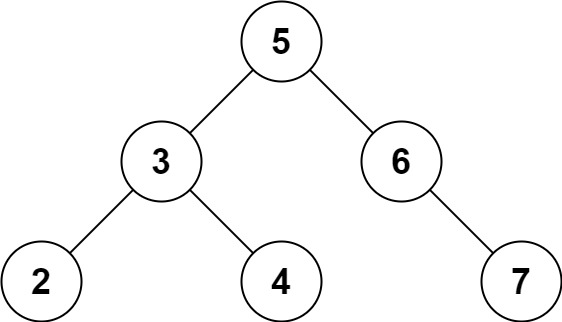## C# || Two Sum IV – How To Get Two Numbers In Binary Search Tree Equal To Target Value Using C#The following is a module with functions which demonstrates how to get two numbers in a binary search tree equal to target value using C#.

1. Find Target – Problem Statement

Given the root of a Binary Search Tree and a target number k, return true if there exist two elements in the BST such that their sum is equal to the given target.

Example 1:``` Input: root = [5,3,6,2,4,null,7], k = 9 Output: true ```

Example 2:``` Input: root = [5,3,6,2,4,null,7], k = 28 Output: false ```

2. Find Target – Solution

The following are two solutions which demonstrates how to get two numbers in a binary search tree equal to target value.

Both solutions use a set to keep track of the items already seen.

Each time a new node is encountered, we subtract the target value from the current node value. If the difference amount from subtracting the two numbers exists in the set, a 2 sum combination exists in the tree

1. Recursive

The following solution uses Depth First Search when looking for the target value.

``` 2. Find Target - Solution - Recursive C# // ============================================================================ // Author: Kenneth Perkins // Date: Oct 9, 2022 // Taken From: http://programmingnotes.org/ // File: Solution.cs // Description: Demonstrates how to get two numbers equal to target value // ============================================================================ public class Solution { public bool FindTarget(TreeNode root, int k) { return Traverse(root, k, new HashSet<int>()); } private bool Traverse(TreeNode node, int k, HashSet<int> seen) { if (node == null) { return false; } // Get remaining value var remaining = k - node.val; // Check if remaining value has been seen if (seen.Contains(remaining)) { return true; } // Add current node value to items seen seen.Add(node.val); // Keep traversing left and right looking for 2 sum return Traverse(node.left, k, seen) || Traverse(node.right, k, seen); } }// http://programmingnotes.org/ 123456789101112131415161718192021222324252627282930 // ============================================================================//    Author: Kenneth Perkins//    Date:   Oct 9, 2022//    Taken From: http://programmingnotes.org///    File:  Solution.cs//    Description: Demonstrates how to get two numbers equal to target value// ============================================================================public class Solution {    public bool FindTarget(TreeNode root, int k) {        return Traverse(root, k, new HashSet<int>());    }     private bool Traverse(TreeNode node, int k, HashSet<int> seen) {        if (node == null) {            return false;        }        // Get remaining value        var remaining = k - node.val;         // Check if remaining value has been seen        if (seen.Contains(remaining)) {            return true;        }        // Add current node value to items seen        seen.Add(node.val);         // Keep traversing left and right looking for 2 sum        return Traverse(node.left, k, seen) || Traverse(node.right, k, seen);    }}// http://programmingnotes.org/ ```

2. Iterative

The following solution uses Breadth First Search when looking for the target value.

``` 2. Find Target - Solution - Iterative C# // ============================================================================ // Author: Kenneth Perkins // Date: Oct 9, 2022 // Taken From: http://programmingnotes.org/ // File: Solution.cs // Description: Demonstrates how to get two numbers equal to target value // ============================================================================ public class Solution { public bool FindTarget(TreeNode root, int k) { if (root == null) { return false; } // Declare stack var stack = new Stack<TreeNode>(); // Keep track of values seen var seen = new HashSet<int>(); // Add root to stack stack.Push(root); // Loop through items on the stack while (stack.Count > 0) { // Get current node var node = stack.Pop(); // Get remaining value var remaining = k - node.val; // Check if remaining value has been seen if (seen.Contains(remaining)) { return true; } else { // Keep traversing left and right looking for 2 sum if (node.left != null) { stack.Push(node.left); } if (node.right != null) { stack.Push(node.right); } } // Add current node value to items seen seen.Add(node.val); } return false; } }// http://programmingnotes.org/ 1234567891011121314151617181920212223242526272829303132333435363738394041424344454647 // ============================================================================//    Author: Kenneth Perkins//    Date:   Oct 9, 2022//    Taken From: http://programmingnotes.org///    File:  Solution.cs//    Description: Demonstrates how to get two numbers equal to target value// ============================================================================public class Solution {    public bool FindTarget(TreeNode root, int k) {        if (root == null) {            return false;        }        // Declare stack        var stack = new Stack<TreeNode>();         // Keep track of values seen        var seen = new HashSet<int>();         // Add root to stack        stack.Push(root);         // Loop through items on the stack        while (stack.Count > 0) {            // Get current node            var node = stack.Pop();             // Get remaining value            var remaining = k - node.val;             // Check if remaining value has been seen            if (seen.Contains(remaining)) {                return true;            } else {                // Keep traversing left and right looking for 2 sum                if (node.left != null) {                    stack.Push(node.left);                }                if (node.right != null) {                    stack.Push(node.right);                }            }            // Add current node value to items seen            seen.Add(node.val);        }        return false;    }}// http://programmingnotes.org/ ```

QUICK NOTES:
The highlighted lines are sections of interest to look out for.

The code is heavily commented, so no further insight is necessary. If you have any questions, feel free to leave a comment below.

Once compiled, you should get this as your output for the example cases:

``` true false ```